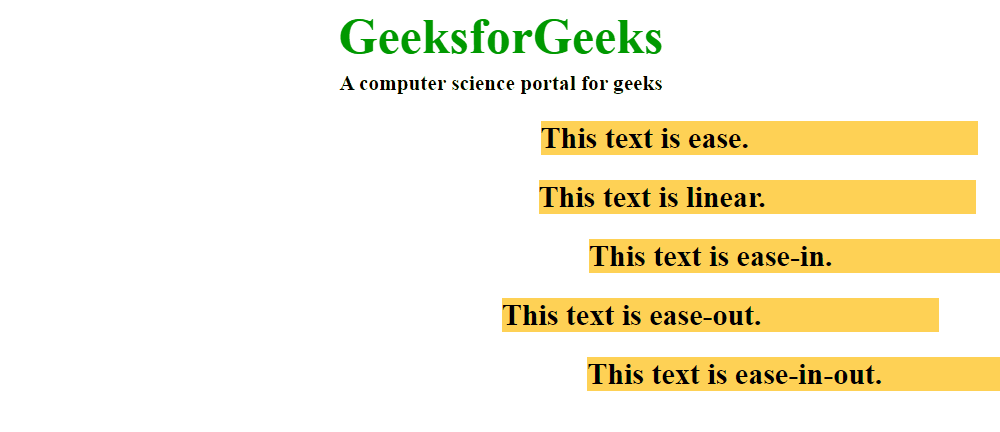Open In App

# CSS animation-timing-function Property

The animation-timing-function property in CSS is used to specify how the animation makes transitions through keyframes. That is, it is used to specify the motion of animation during transitions.

Syntax:

`animation-timing-function: linear | ease | ease-in | ease-out | ease-in-out |step-start | step-end|steps(int, start | end) | cubic-bezier(n, n, n, n) | initial | inherit;`

Property Value:

• ease: With this property value, the animation starts slowly, then fast, and then finally ends slowly (this is default).
• linear: If this value is specified for the property then the animation plays with the same speed from start to end.
• ease-in: If this value is specified then the animation begins with a slow start.
• ease-out: If this value is specified for the property then the animation plays normally but ends slow. This is similar to ease-in.
• ease-in-out: With this property value, the animation both starts and ends slow.

Example: HTML Program to illustrate the above property values for the animation-timing-function property.

## HTML

 ```<``html``>``  ` `<``head``>``    ``<``title``>``        ``CSS | animation-timing-function Property``    ````    ``<``style``>``        ``.geeks {``            ``font-size: 40px;``            ``text-align: center;``            ``font-weight: bold;``            ``color: #090;``            ``padding-bottom: 5px;``            ``font-family: Times New Roman;``        ``}` `        ``.geeks1 {``            ``font-size: 17px;``            ``font-weight: bold;``            ``text-align: center;``            ``font-family: Times New Roman;``        ``}` `        ``h2 {``            ``width: 350px;``            ``animation-name: text;``            ``animation-duration: 4s;``            ``animation-iteration-count: infinite;``            ``background-color: rgb(255, 210, 85);``        ``}` `        ``#one {``            ``animation-timing-function: ease;``        ``}` `        ``#two {``            ``animation-timing-function: linear;``        ``}` `        ``#three {``            ``animation-timing-function: ease-in;``        ``}` `        ``#four {``            ``animation-timing-function: ease-out;``        ``}` `        ``#five {``            ``animation-timing-function: ease-in-out;``        ``}` `        ``@keyframes text {``            ``from {``                ``margin-left: 60%;``            ``}` `            ``to {``                ``margin-left: 0%;``            ``}``        ``}``    ````` `<``body``>``    ``<``div` `class``=``"geeks"``>``        ``GeeksforGeeks``    ````    ``<``div` `class``=``"geeks1"``>``        ``A computer science portal for geeks``    ````    ````    ``<``h2` `id``=``"one"``>``        ``This text is ease.``    ````    ````    ``<``h2` `id``=``"two"``>``        ``This text is linear.``    ````    ````    ``<``h2` `id``=``"three"``>``        ``This text is ease-in.``    ````    ````    ``<``h2` `id``=``"four"``>``        ``This text is ease-out.``    ````    ````    ``<``h2` `id``=``"five"``>``        ``This text is ease-in-out.``    ``````  ` ``

Output:Supported Browser: The browser supported by animation-timing-function property are listed below:

• Google Chrome 43.0 and above
• Edge 12.0 and above
• Firefox 16.0 and above
• Opera 30.0 and above
• Safari 9.0 and above